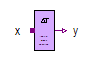Reference Voltage Source - MapleSim Help

Quasistationary Multiphase Reference Voltage Source

Variable multiphase AC voltage with reference angle inputDescription The Quasistationary Multiphase Reference Voltage Source (or Reference Voltage Source) component contains $m$ quasistationary single-phase voltage source models, each connected between corresponding phases of ${\mathrm{plug}}_{p}$ and ${\mathrm{plug}}_{n}$, with the voltages set by the complex vector input $I$ and the reference angle set by the real input, $\mathrm{\gamma }$.Equations $i={i}_{p}=-{i}_{n}$ $v={v}_{p}-{v}_{n}=V$ $\mathrm{\omega }=\frac{d{\mathrm{\gamma }}_{p}}{\mathrm{dt}}$ $\mathrm{\gamma }={\mathrm{\gamma }}_{p}={\mathrm{\gamma }}_{n}$Connections

 Name Description Modelica ID ${\mathrm{plug}}_{p}$ Positive quasistationary multiphase plug plug_p ${\mathrm{plug}}_{n}$ Negative quasistationary multiphase plug plug_n $\mathrm{\gamma }$ Reference angle of voltage source gamma $V$ Complex $m$-vector input; voltage in [V] VParameters

 Name Default Units Description Modelica ID $m$ $3$ Number of phases mModelica Standard Library The component described in this topic is from the Modelica Standard Library. To view the original documentation, which includes author and copyright information, click here.